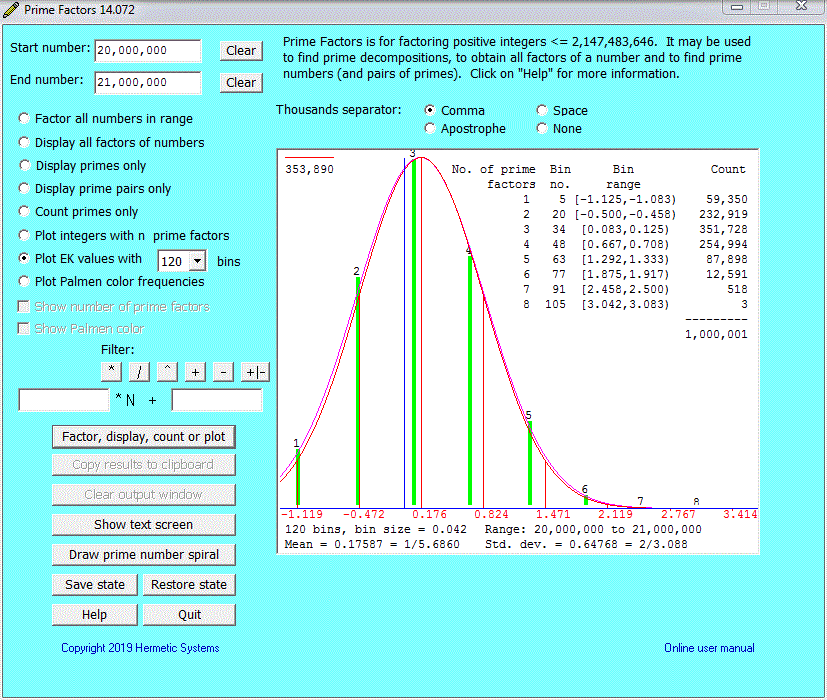Prime Factors Plotting the Erdös-Kac Histograms

The starting point here is the web page by Matthew Watkins entitled the Erdös-Kac theorem, where we read that the numbers of prime factors of large integers (suitably normalised) tend to follow the Gaussian distribution. This web page should be read for a deeper understanding of the significance of the Erdös-Kac histograms produced by the Prime Factors software.

Matthew Watkins speaks of the numbers of prime factors of large integers "suitably normalised". By this he refers to the values, here called "EK values", defined as:

```NPF(n) - log(log(n))
--------------------
sqrt(log(log(n)))```

where NPF(n) is the number of prime factors of n, log() is the natural logarithm and sqrt() is the square root.

Suppose we consider a range of integers and calculate the EK value for each integer. The range of EK values for numbers in the range 3 through 231 is -1.180 through 3.515 (the EK value for 2 is undefined), so we can divide this range of EK values into k "bins" and assign the calculated values to their appropriate bins, then count how many values are contained in each bin and plot a histogram. The Prime Factors software will do this for us, and we obtain results such as:For more information see the page on the ErdösKac theorem at Wikipedia.